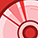Announcements

#### Curious on how you can apply Rador Sensors to Smart Home Appliances? Register for the ASEAN Smart Home Appliance Webinar here to find out how.

Tip / Sign in to post questions, reply, level up, and achieve exciting badges. Know more

# Radar sensor Forum DiscussionsLevel 2Level 2

# Position2Go Raw data in DAVE IDE

Hello,

I develop my own algorithm.And I have a question about raw data.

Getting the raw data seems to be using frame_info->sample_data

Is to use uint16_t radar_get_frame(Radar_Handle_t device, Frame_Info_t* frame_info, uint8_t wait_for_data) in radar_base.c?

And how can I achieve complex style like matlab paradigm. (ex: 0.5008547 + 0.5177045*i)

Is sample_data = sample_data(1:frame_info.num_samples_per_chirp,:,:) + 1i * sample_data((frame_info.num_samples_per_chirp+1):end,:,:); complete the complex style in matlab?

Because my algorithm is based on matlab,so I need the raw data in complex style

Please reply back with how to get complex raw data like matlab in DAVE IDE.

Thanks

1 SolutionModeratorModerator

# Re: Position2Go Raw data in DAVE IDE

Hi @_Tom_ ,

For the first part, the fft_input_i1[i]fft_input_q1[i] as seen in XMC_DEBUG()_printf.png, store the ADC values, which are not normalized, as you see in example of extract_raw_data.c or MATLAB example.
To normalize the values just divide it by 4096, since the ADC has 12-bit resolution.
Sorry for the confusion.

For the second part to extract the Q data in the example_raw_data.c in Comm_lib_interface please go through the following thread -link

Thanks and regards,
Yashraj

5 RepliesModeratorModerator

# Re: Position2Go Raw data in DAVE IDE

HI @_Tom_ ,

For getting the raw data in DAVE project, the function you referred does the job.
And the second function in parseMSG_FRAME_DATA.m file does the job of parsing the raw data in complex style, if that is the chosen format.

The current P2G_FMCW DAVE project captures both I and Q samples, by setting the signal_part in frame_format as RADAR_SIGNAL_I_AND_Q = 2 in Signal_Part_t struct. It is already in the complex style.

Thanks and regards,
YashrajLevel 2Level 2

# Re: Position2Go Raw data in DAVE IDE

Hi @PalY

Yes,RADAR_SIGNAL_I_AND_Q = 2 is already in the complex style for matlab.

It is setting by frame_format,but I want to write complex style in DAVE_project/Algorithm/my_algorithm.c

It's like P2G_FMCW/Algorithm/range_doppler.c

fft_input_i1[i] = range_fft_signal_rx1[idx];
fft_input_q1[i] = range_fft_signal_rx1[idx + 1];

But I think   " fft_input_i1[i] + fft_input_q1[i]*I "  is not equal to " sample_data = sample_data(1:frame_info.num_samples_per_chirp,:,:) + 1i * sample_data((frame_info.num_samples_per_chirp+1):end,:,:);  ".

I use XMC_DEBUG() to printf  " fft_input_i1[i] + fft_input_q1[i] ".

It print int style,not float, double or complex style.

So I want to ask is about how to get raw gata for complex style in DAVE_project/Algorithm/my_algorithm.c

Thank you for your helpModeratorModerator

# Re: Position2Go Raw data in DAVE IDE

Hi @_Tom_ ,

As I can see in the screenshot, the p_temp are holding the Q2 channel's ADC values, and you are converting the value to uint16, loosing the decimal info. Even if you typecast the values to float, it will not have that info, that is why it is printing zeros after the decimal point in print statements.
Are you doing the same for p_temp and p_temp?
If that is the case could you try not typecasting in first place or typecasting to floating-pointer?

Well for the complex style the 'I' represents the real values and 'Q' represents the imaginary part. Since, you are able to extract I and Q data,
fft_input_i1[i] + fft_input_q1[i]*I "   = "sample_data(1:frame_info.num_samples_per_chirp,:,:) + 1i * sample_data((frame_info.num_samples_per_chirp+1):end,:,:);".

Thanks and regards,
YashrajLevel 2Level 2

# Re: Position2Go Raw data in DAVE IDE

Hi @PalY ,

I try not typecasting in first place or typecasting to floating-pointer.

Using XMC_DEBUG() to printf value still like the picture XMC_DEBUG()_printf.png .

uint16_t *p_temp;

p_temp = (uint16_t *)get_buffer_address_by_chirp(p_acq_buf, 0, j );
p_temp = (uint16_t *)get_buffer_address_by_chirp(p_acq_buf, 1, j );

fft_input_i1[i] = range_fft_signal_rx1[idx];
fft_input_q1[i] = range_fft_signal_rx1[idx + 1];

This is the reference range_doppler.c algorithm in P2G_FMCW.

It is printing zeros after the decimal point in print statements. I think it's because p_acq_buf  type is uint8_t.T hen it typecast the values to float,it still printing zeros after the decimal point.

Could use like ComLib_C_Interface\examples\extract_raw_data.c

// Print the sampled data which can be found in frame_info->sample_data
for (uint32_t i = 0; i < frame_info->num_samples_per_chirp; i++){printf("ADC sample %d: %f\n", i, frame_info->sample_data[i]);}

using frame_info->sample_data[i] to get raw data?

And ComLib_C_Interface\examples\extract_raw_data.c ADC sample only print  64 points real sample_data.

How do I revise,then the ADC sample can print real and imag sample_data.

Thanks.ModeratorModerator

# Re: Position2Go Raw data in DAVE IDE